# Percent and ProportionsIn previous lessons, you were shown how to convert a decimal to a percent and a percent to a decimal. Thus, if you were asked to Find 15% of 120, you would multiply .15 by 120, to get an answer of 18. But what would you do if you given this problem: 8 is what percent of 20? In this problem, the percent is the unknown quantity! We need to figure out how to find this unknown quantity.

Every statement of percent can be expressed verbally as: "One number is some percent of another number." Percent statements will always involve three numbers. For example:

____ is ____ % of ____.

In the problem, 8 is what percent of 20?, the number 8 is some percent of the number 20. Looking at this problem, it is clear that 8 is the part and 20 is the whole. Similarly, in the statement, "One number is some percent of another number.", the phrase "one number" represents the part and "another number" represents the whole. Thus the statement, "One number is some percent of another number.", can be rewritten:

"One number is some percent of another number.", becomes, "The part is some percent of the whole."

From previous lessons we know that the word "is" means equals and the word "of" means multiply. Thus, we can rewrite the statement above:

The statement: "The part is some percent of the whole.", becomes the equation:

the part = some percent x the whole

Since a percent is a ratio whose second term is 100, we can use this fact to rewrite the equation above as follows:

the part = some percent x the whole

becomes:

the part =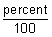x the whole

Dividing both sides by "the whole" we get the following proportion: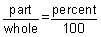Since percent statements always involve three numbers, given any two of these numbers, we can find the third using the proportion above. Let's look at an example of this.Problem 1: If 8 out of 20 students in a class are boys, what percent of the class is made up of boys?

Analysis: In this problem, you are being asked 8 is what percent of 20? You are given two numbers from the proportion above and asked to find the third. The percent is the unknown quantity in this problem. We need to find this unknown quantity.

Identify: The phrase 8 is means that 8 is the part.

The phrase what percent tells us that percent is the unknown quantity. This unknown quantity will be represented by x in our proportion.

The phrase of 20 means that 20 is the whole.

Substitute: Now we can substitute these values into our proportion.becomesSolve: Cross multiply and we get:  20x  = 800

Divide both sides by 20 to solve for x and we get:  x = 40

Solution: 8 is 40% of 20. Therefore, 40% of the class is made up of boys.

Note that in Problem 1 we did not have to cross multiply to solve the proportion. We could have used equivalent fractions instead (i.e., since 20 multiplied by 5 equals 100, we get that 8 multiplied by 5 equals x, so x equals 40).

In Problem 1 we were asked 8 is what percent of 20? and we found the solution by substituting into a proportion. But how would we solve this problem: 18 is 40% of what number? and how would we solve this problem: What is 20% of 45? We will look at these last two problems below.

Problem 2: 18 is 40% of what number?

Identify: The phrase 18 is means that 18 is the part.

40% means that 40 will replace percent in our proportion.

The phrase of what number represents the whole and is the unknown quantity. We will let variable x represent this unknown quantity in our proportion.

Substitute: Now we can substitute these values into our proportion.becomes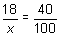Solve: Cross multiply and we get:  40x  = 18(100) or 40x  = 1800

Divide both sides by 40 to solve for x  and we get:  x  = 45

Solution: 18 is 40% of 45

Problem 3: What is 20% of 45?

Identify: The phrase what is means represents the part and is the unknown quantity. We will let variable x represent this unknown quantity in our proportion.

20% means that 20 will replace percent in our proportion.

The phrase of 45 means that 45 is the whole.

Substitute: Now we can substitute these values into our proportion.becomesSolve: Cross multiply and we get:  100x  = 45(20) or 100x  = 900

Divide both sides by 100 to solve for x  and we get:  x  = 9

Solution: 9 is 20% of 45

In Problems 1, 2 and 3 we are given two numbers and asked to find the third by using a proportion. However, the unknown quantity was different for each problem. Let's compare these problems in the table below. Red is used for the unknown quantity in each problem.

 Problem 1 Problem 2 Problem 3 statement 8 is what percent of 20? 18 is 40% of what number? What is 20% of 45? part 8 18 x = What is percent x = what percent 40% 20% whole 20 x = of what number 45

In Problem 1 we let x represent the unknown quantity "what percent"; in Problem 2 we let x represent the unknown quantity "of what number"; and in Problem 3 we let x represent the unknown quantity "What is." Thus, we solved three different percent problems, where in each problem, two numbers were given and we were asked to find the third. We did this by letting a variable represent the unknown quantity and then substituting the given values into a proportion to solve for the unknown quantity.

Note that in all three percent statements, the whole always follows the word "of" and the part always precedes the word "is". This is not surprising since our original statement is, "One number is some percent of another number." Thus, we can revise our proportion as follows:becomesLet's solve some more percent problems using proportions.

Problem 4: What is 25% of 52?

Identify: 25% means that 25 will replace PERCENT in our proportion.

52 is the whole and will replace OF in our proportion.

The part is the unknown quantity and will be represented by p in our proportion.

Substitute: Now we can substitute these values into our proportion.becomes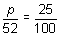Solve: Cross multiply and we get:  100p = 52(25) or 100p = 1300

Divide both sides by 100 to solve for p and we get:  p = 13

Solution: 13 is 25% of 52

Note that we could restate this problem as, "Find 25% of 52", and get the same answer. However, in the interest of consistency, we will use proportions to solve percent problems throughout this lesson. In Problems 5 through 7, we will use to represent the unknown quantity.

Problem 5: What percent of 56 is 14?

Identify: 56 is the whole and will replace OF in our proportion.

14 is the part and will replace IS in our proportion.

PERCENT is the unknown quantity in our proportion, to be represented by n.

Substitute:becomesSolve: Cross multiply and we get:  56n = 14(100), or 56n = 1400

Divide both sides by 56 and we get:  n = 25

Solution: 25% of 56 is 14

Problem 6: 18 is 75% of what number?

Identify: 18 is the part and will replace IS in our proportion.

75% means that 75 will replace PERCENT in our proportion.

The whole is the unknown quantity in our proportion, to be represented by n.

Substitute:becomes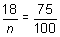Solve: Cross multiply and we get:  75= 18(100) or 75n = 1800

Divide both sides by 75 and we get:  n = 24

Solution: 18 is 75% of 24

Problem 7: What is 15% of 200?

Identify: 15% means that 25 will replace PERCENT in our proportion.

200 is the whole and will replace OF in our proportion.

The part is the unknown quantity in our proportion, to be represented by n

Substitute:becomes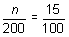Solve: Cross multiply and we get:  100n = 200(15) or 100= 3000

Divide both sides by 100 and we get:  n = 30

Solution: 30 is 15% of 200

Now that we have solved a number of percent problems using proportions, we can go back to the type of problem presented at the beginning of this lesson: In Problems 8 through 10 we will solve real world problems, using different variables to represent the unknown quantity in each problem.Problem 8: At Little Rock School, 476 students ride their bike to school. If this number is 85% of the school enrollment, then how many students are enrolled?

Identify: This problem can be rewritten as 476 is 85% of what number?

476 is the part and will replace IS in our proportion.

The percent given is 85%.

The whole is the unknown quantity, so y will represent the OF in our proportion.

Substitute: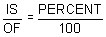becomes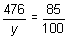Solve: Cross multiply and we get:  85y = 47600

Divide both sides by 85 and we get:  y = 560

Solution: There are 560 students enrolled at Little Rock School.Problem 9: A football team won 75% of 120 games in a season. How many games is that?

Identify: This problem can be rewritten as What is 75% of 120?

120 is the whole and will replace the OF in our proportion.

The percent given is 75%.

The part is the unknown quantity, so p will represent the IS in our proportion.

Substitute:becomes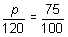Solve: Cross multiply and we get:  100p = 9000

Divide both sides by 85 and we get:  p = 90

Solution: The team won 90 games.Problem 10: Jennie has \$300 and she spends \$15. What percent of her money is spent?

Identify: This problem can be rewritten as \$15 is what percent of \$300?

15 is the part and will replace the IS in our proportion.

300 is the whole and will replace the OF in our proportion.

Percent is the unknown quantity, so will represent the PERCENT in our proportion.

Substitute:becomes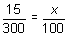Solve: Cross multiply and we get:  300x = 1500

Divide both sides by 300 and we get:  x = 5

Solution: Jennie spent 5% of her money.

Summary: Every statement of percent can be expressed verbally as: "One number is some percent of another number." Percent statements will always involve three numbers. Given two of these numbers, we can find the third by substituting into one of the proportions below.ORIn this lesson, we solved percent problems using proportions by following this procedure:

2. Identify what information is given.
3. Identify what information is unknown.
4. Use a variable to represent the unknown quantity.
5. Set up a proportion for the problem by substituting the given information and the variable into one of the proportions listed above.
6. Evaluate and solve the proportion in Step 5 to find the unknown quantity.

### Exercises

Directions: Solve each percent problem below using a proportion. If your answer is a percent, do NOT enter the percent symbol. Just enter the number. For each exercise below click once in the ANSWER BOX and then type in your answer; then click ENTER. Your answers should be given as whole numbers greater than zero. After you click ENTER, a message will appear in the RESULTS BOX to indicate whether your answer is correct or incorrect. To start over, click CLEAR.

 1.What is 20% of 90? ANSWER BOX:    RESULTS BOX:
 2.14 out of 56 students got an A. What percent got an A? ANSWER BOX:  %  RESULTS BOX:
 3.There are 18 girls in a class. If this is 40% of the class list, then how many students are in the class? ANSWER BOX:    RESULTS BOX:
 4.In a school 25% of 312 students bring lunch from home. How many students is that? ANSWER BOX:    RESULTS BOX:
 5.12 out of 30 students studied Spanish. What percent studied Spanish? ANSWER BOX:  %  RESULTS BOX: